Ex 12.2

Chapter 12 Class 7 Symmetry
Serial order wise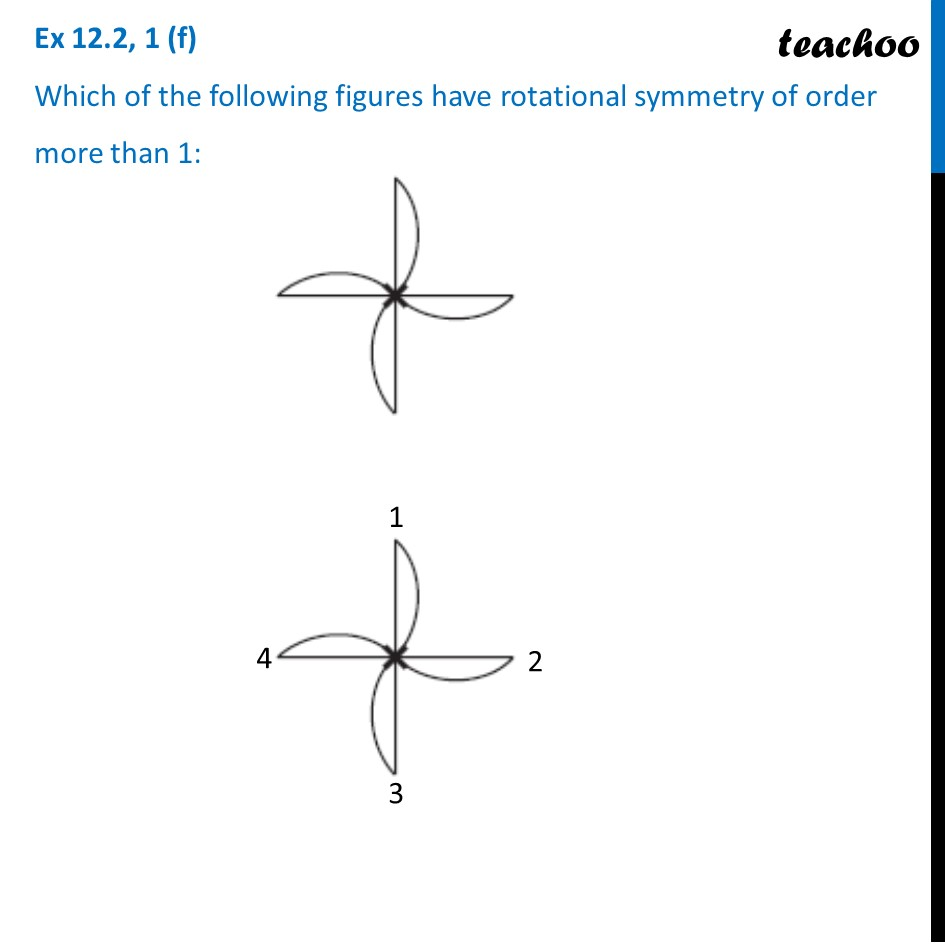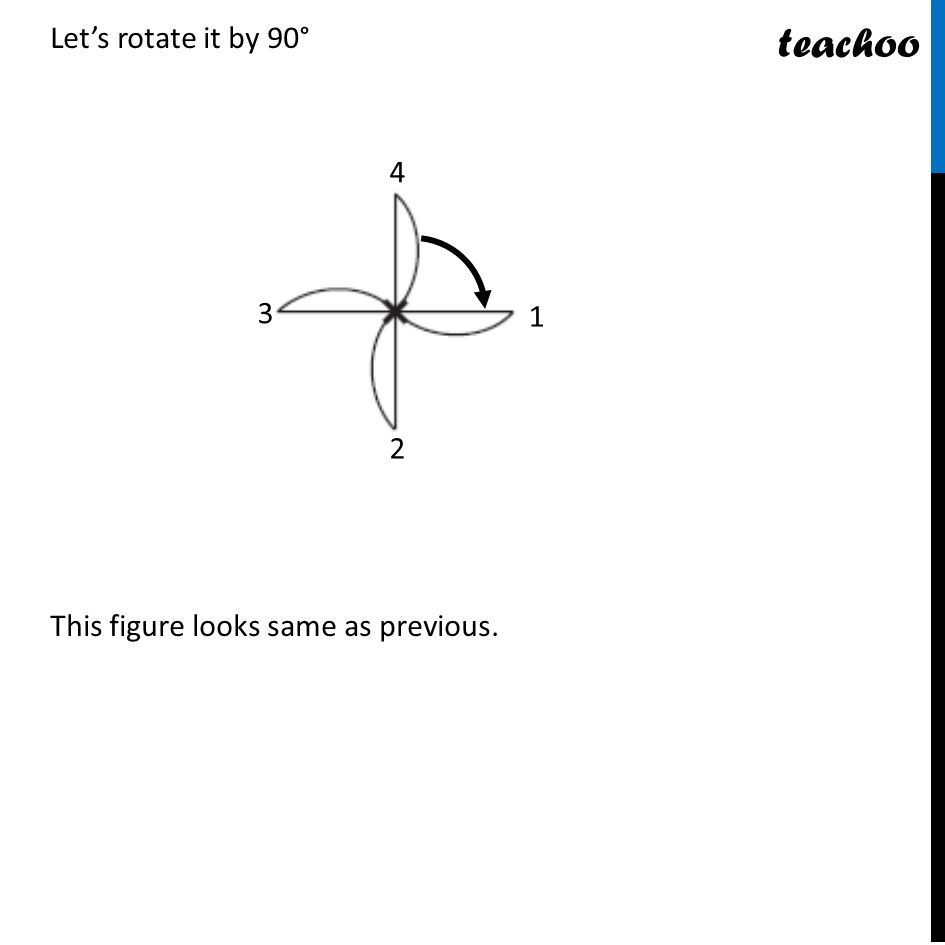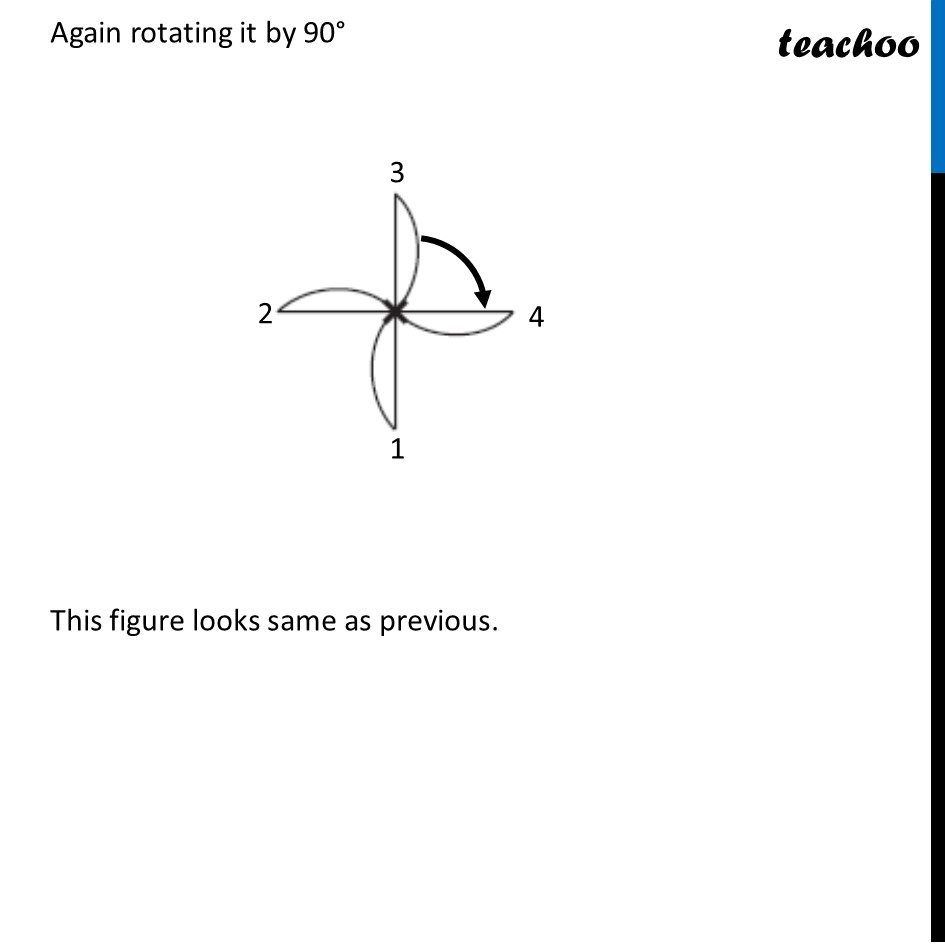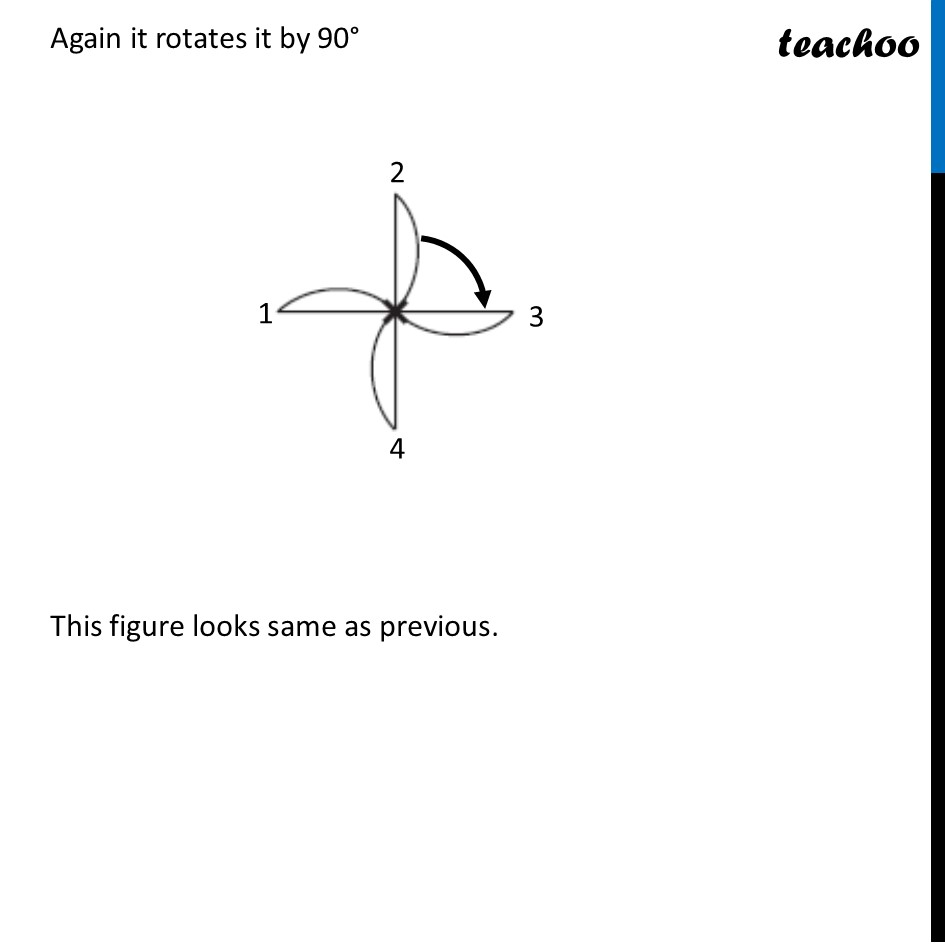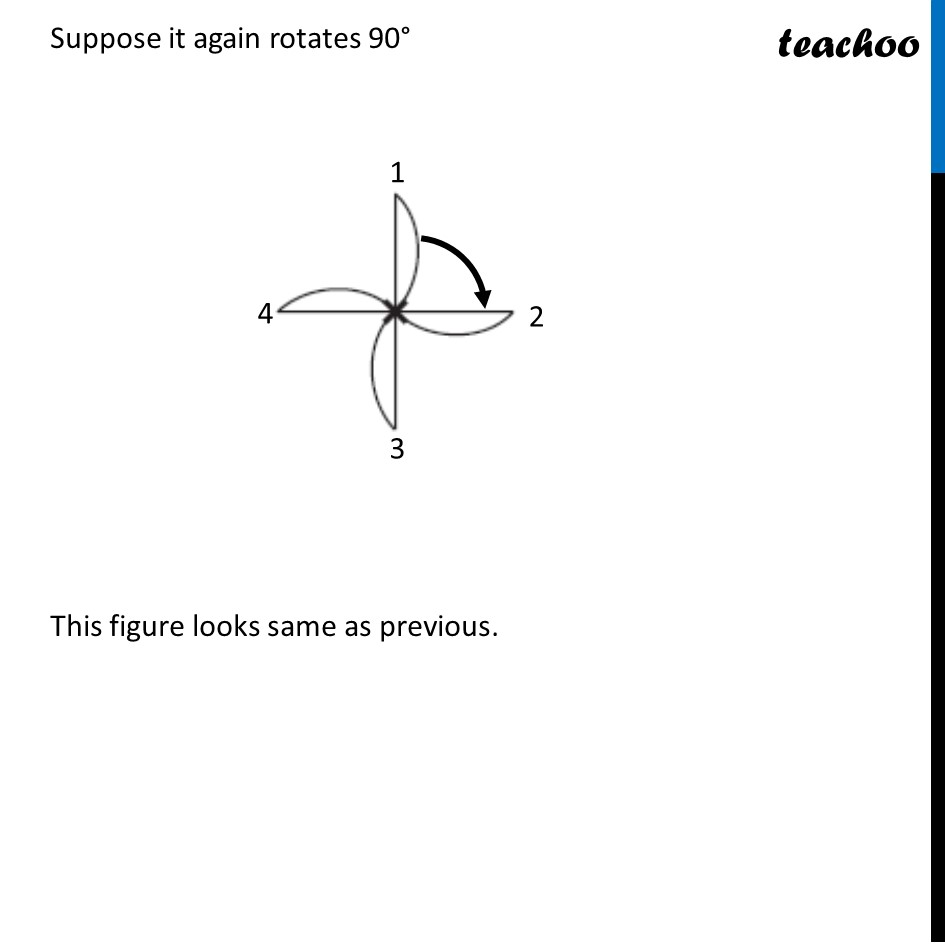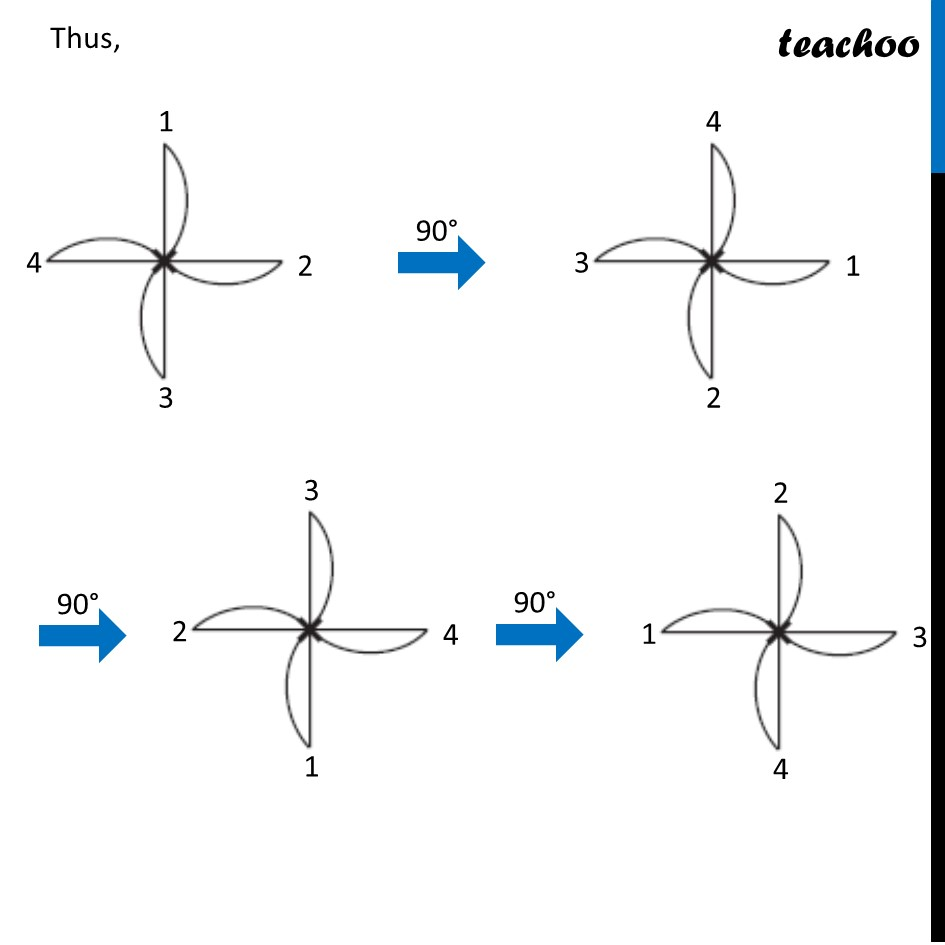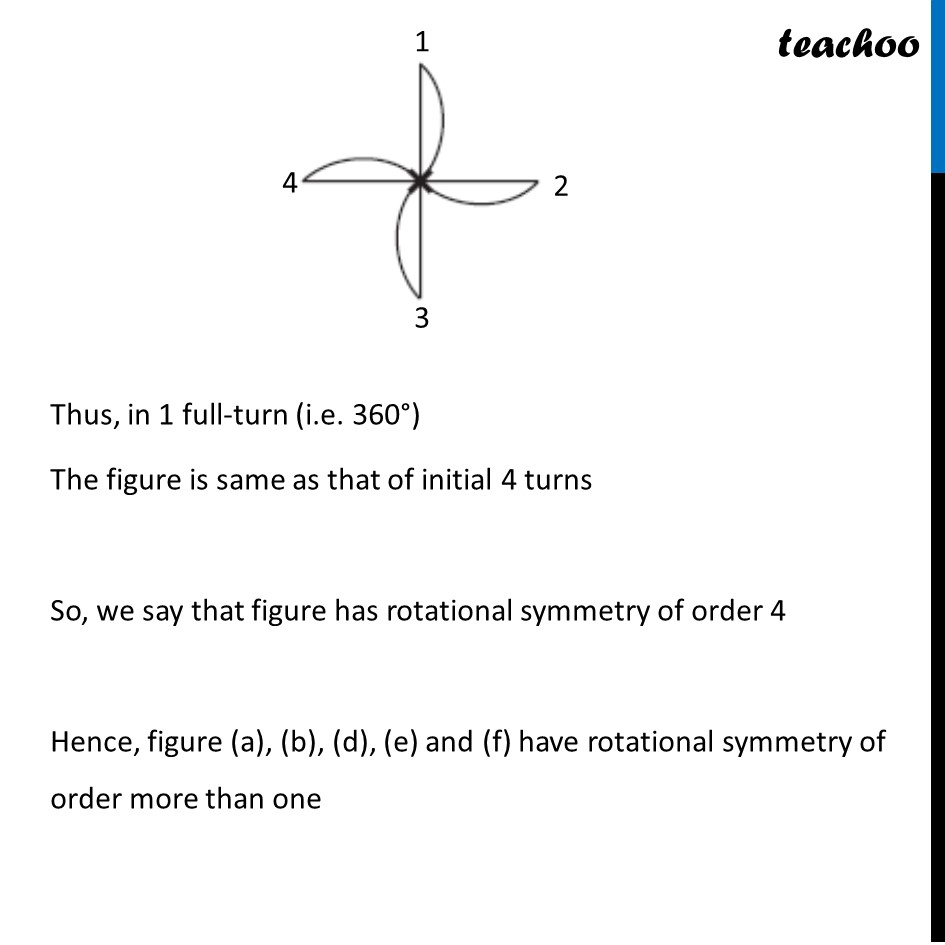Learn in your speed, with individual attention - Teachoo Maths 1-on-1 Class

### Transcript

Ex 12.2, 1 (f) Which of the following figures have rotational symmetry of order more than 1: Let’s rotate it by 90° This figure looks same as previous. Again rotating it by 90° This figure looks same as previous. Again it rotates it by 90° This figure looks same as previous. Suppose it again rotates 90° This figure looks same as previous. Thus, in 1 full-turn (i.e. 360°) The figure is same as that of initial 4 turns So, we say that figure has rotational symmetry of order 4 Hence, figure (a), (b), (d), (e) and (f) have rotational symmetry of order more than one